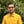Trusted answers to developer questions
Trusted Answers to Developer Questions

Related Tags

scala
math
sqrt
communitycreator

# What is Math.sqrt() in Scala?Harris Amjad

Grokking Modern System Design Interview for Engineers & Managers

Ace your System Design Interview and take your career to the next level. Learn to handle the design of applications like Netflix, Quora, Facebook, Uber, and many more in a 45-min interview. Learn the RESHADED framework for architecting web-scale applications by determining requirements, constraints, and assumptions before diving into a step-by-step design process.

The sqrt() function returns the square root of a number sent as a parameter.

Figure 1 below shows the mathematical representation of the sqrt() function.

Figure 1: Mathematical representation of the sqrt() function

The following module is required for this function:

import scala.math._


### Syntax

Double sqrt(Double number)


### Parameter

This function requires a number as a parameter.

### Return value

• If the input is a valid positive double number, then the square root of the number sent as a parameter is returned.
• If the parameter value is positive infinity, then it returns positive infinity.
• If the parameter value is NaN, less than zero, or negative infinity, then it returns NaN.

### Example

import scala.math._object Main extends App {    //positive number    println(s"The value of sqrt(10) = ${sqrt(10)}"); println(s"The value of sqrt(0) =${sqrt(0)}");     println(s"The value of sqrt(4) = ${sqrt(4)}"); //error outputs println(s"The value of sqrt(Double.PositiveInfinity) =${sqrt(Double.PositiveInfinity)}");    println(s"The value of sqrt(Double.NegativeInfinity) = ${sqrt(Double.NegativeInfinity)}"); println(s"The value of sqrt(Double.NaN) =${sqrt(Double.NaN)}");    println(s"The value of sqrt(-1) = \${sqrt(-1)}");     }

RELATED TAGS

scala
math
sqrt
communitycreator

CONTRIBUTORHarris Amjad

Grokking Modern System Design Interview for Engineers & Managers

Ace your System Design Interview and take your career to the next level. Learn to handle the design of applications like Netflix, Quora, Facebook, Uber, and many more in a 45-min interview. Learn the RESHADED framework for architecting web-scale applications by determining requirements, constraints, and assumptions before diving into a step-by-step design process.

Keep Exploring

Learn in-demand tech skills in half the time